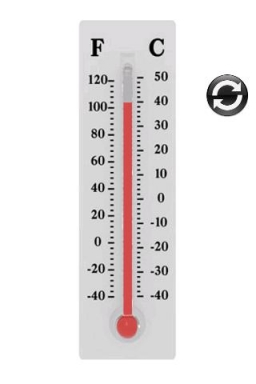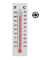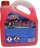# The temperature 9

The temperature at 9 AM is 2 degrees. The temperature rises 3 more degrees by noon. Which expression describes the temperature at noon? Calculate the new temperature value.

t =  5 °

### Step-by-step explanation:

$t=2+3=5\text{°}$Did you find an error or inaccuracy? Feel free to write us. Thank you!

Showing 1 comment:Cutnessnkills11
i am having a great time using this fraction calculator## Related math problems and questions:

• Temperature up and dowmAt 6 AM, the temperature is 19.4°C. Between 6 AM and noon, the temperature rises 3.8°C. Between noon and 6 PM, the temperature falls 2.5°C. What is the temperature at 6 PM?
• ThermometerThe thermometer showed -12 degrees Celsius in the morning then the temperature rises by 4 degrees and later again increased by 2 degrees at the evening has fallen by 5 degrees and then falls 3 degrees. What end temperature does the thermometer show?At a weather centre, the temperature at midnight was -2 degree Celsius and by noon it had raised 4 degree Celsius. What is the new temperature?
• OpheliaOphelia recorded the temperature of a cold store every two hours. 1. At 6 am, it was -4°C, and at 8 am, it was -1°C. By how much did the temperature rise? 2. The temperature went up by 5°C in the next two hours. .What was the temperature at 10 am?
• Outside temperatureThe temperature outside was 57 degree Fahrenheit. During the next few hours it decreased by 18 degrees and then increased by 23 degrees. Find new temperature.
• At noon 2At noon, the temperature was 4 degrees fahrenheit. At 6pm, it was 10 degrees colder. What was the temperature at 6pm?
• The temperatureThe temperature at 1:00 was 10 F. Between 1:00 and 2:00, the temperature dropped 15F. Between 2:00 and 3:00, the temperature rose 3F. What is the temperature at 3:00?
• Temperature variationsToday's temperature was 80 degrees, and then the temperature dropped 10 degrees. Then it dropped 15 degrees again, then the next day, the temperature went up 2 degrees. What would the temperature be?
• This morningThis morning it was 80 degrees F outside the temperature increased 12 degrees F. What is the temperature now?
• Temperature change 3At 2 PM, The temperature was 76 degrees Fahrenheit. At 8 PM, the temperature was 58 degrees Fahrenheit. What was the change in temperature?
• WeatherWeather. At 5:00 am, the temperature was 28°C, and at noontime, it rose by 9°C. By 4:00 pm, it dropped 3°C, and by nighttime, it was 4°C less. What is the room temperature this time?
• The temperature 8The temperature on Monday was –6°C. On Tuesday, the temperature was 3 degrees lower. Write down the temperature on Tuesday.
• The temperature 10The temperature in a freezer is -15°C, and it increases by 3°C.
• Outdoor temperatureThe outdoor temperature was 8 degrees at midnight. The temperature declined 5 degrees during each of the next 3 hours. What was the temperature at 3am?
• Temperature 2Sunday's high temperature was 3 degrees higher than Saturday's. On Monday, the temperature fell 5 degrees, then rose 7 degrees on Tuesday and 4 more on Wednesday. Then it fell 17 degrees to a record low of 31 on Thursday. What was the temperature on Satur
• The temperature 3The temperature is -12 degrees Fahrenheit. During the following 5 hours, the temperature decrease by 23 degrees Fahrenheit. What is the weather at 11 pm?
• Expression plus minusEvaluate expression: (-1)2 . 12 – 6 : 3 + (-3) . (-2) + 22 – (-3) . 2